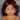# int x=5; x += x++ + ++x; (C#)

January 04, 2019

I had this question as my WhatsApp status and got interesting answers from friends. I will explain the answer here.

#### increment and decrement operators are done differently in C# and C

One thing that you have to remember is, the way C# does it is different than how C does it. Many people reason as though C# and C are the same language; they are not.

here is the great explanation about c# pre and post increment operators.

#### Explanation

``````int x = 5;
x += x++ + ++x;``````
##### Step 0
``x += x++ + ++x;``
##### Step 1
``x = x + (x++) + (++x);``
##### Step 2
``x = 5 + (x++) + (++x);``
##### Step 3
``x = 5 + 5 + (++x);``

step #3 is very important. x++ result is 5, but the variable ‘x’ value has been incremented to 6. so the next ‘x’ value is 6. Read this link for more explanation.

##### Step 4
``````x = 5 + 5 + (++6)
= 5 + 5 + 7
= 17``````

so answer for int x=5; x += x++ + ++x; is 17Personal website and blog by M Kumaran